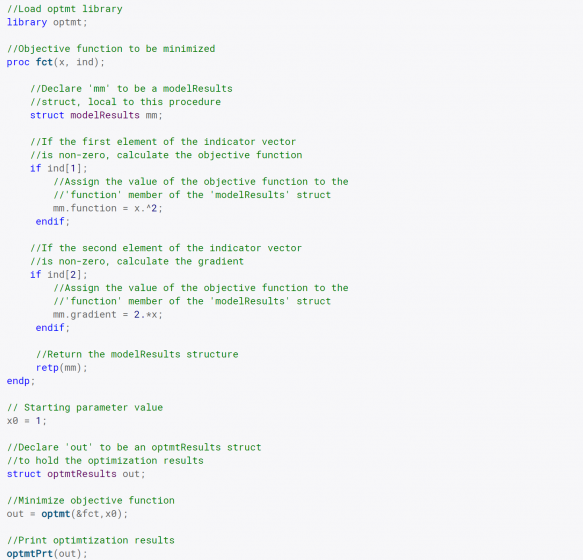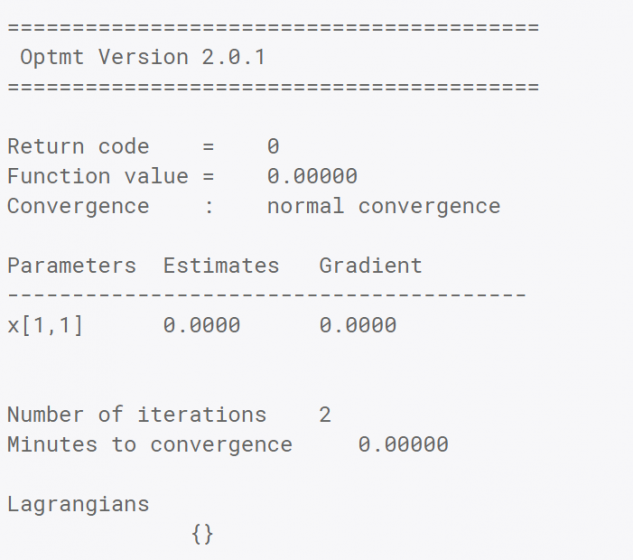OPMT is intended for the optimization of functions. It has many features, including a wide selection of descent algorithms, step-length methods, and “on-the-fly” algorithm switching. Default selections permit you to use Optimization with a minimum of programming effort. All you provide is the function to be optimized and start values, and OPMT does the rest.

Version 2.0 is easier to use than ever!

New syntax options eliminate the need for PV and DS structures:

Decreasing the required code up to 25%.

Decreasing runtime up to 20%.

Simplifying usage.

Optional dynamic arguments make it simple and transparent to add extra data arguments beyond model parameters to your objective function.

Updated documentation and examples.

Fully backwards compatible with OPTMT 1.0

Key Features

Descent methods

BFGS (Broyden, Fletcher, Goldfarb and Powell)

DFP (Davidon, Fletcher and Powell)

Newton

Steepest Descent

Line search methods

STEPBT

Brent’s method

HALF

Strong Wolfe’s Conditions New!

Flexible

Bounded parameters.

Specify fixed and free parameters.

Dynamic algorithm switching.

Compute all, a subset, or none of the derivatives numerically.

Easily pass data other than the model parameters as extra input arguments. New!

Efficient

Option to avoid computations that are the same for the objective function and derivatives.

The tremendous speed of user-defined procedures in GAUSS speeds up your optimization problems.

Trusted

For more than 30 years, leading researchers have trusted the efficient and numerically sound code in the GAUSS optimization packages to keep them at the forefront of their fields.

Details

Novice users will typically leave most of these options at the default values. However, they can be a great help when tackling more difficult problems.

Control options
Parameter bounds Simple parameter bounds of the type: lower_bd ≤ x_i ≤ upper_bd
Descent algorithms BFGS, DFP, Newton and Steepest Descent.
Algorithm switching Specify descent algorithms to switch between based upon the number of elapsed iterations, a minimum change in the objective function or line search step size.
Line search method STEPBT (quadratic and cubic curve fit), Brent’s method, half-step or Strong Wolfe’s Conditions.
Active parameters Control which parameters are active (to be estimated) and which should be fixed to their start value.
Gradient Method Either compute an analytical gradient, or have OPTMT compute a numerical gradient using the forward, central or backwards difference method.
Hessian Method Either compute an analytical Hessian, or have OPTMT compute a numerical Hessian using the forward, central or backwards difference method.
Gradient check Compares the analytical gradient computed by the user supplied function with the numerical gradient to check the analytical gradient for correctness.
Random seed Starting seed value used by the random line search method to allow for repeatable code.
Print output Controls whether (or how often) iteration output is printed and whether a final report is printed.
Gradient step Advanced feature: Controls the increment size for computing the step size for numerical first and second derivatives.
Maximum iterations Maximum iterations to converge.
Maximum elapsed time Maximum number of minutes to converge.
Maximum random search attempts Maximum allowed number of random line search attempts.
Convergence tolerance Convergence is achieved when the direction vector changes less than this amount.

The code below finds the minimum of the simple function x2. The objective function in this case computes the function value and/or the gradient, depending upon the value of the incoming indicator vector, ind. This makes it simple to avoid duplication of calculations which are required for the objective function and the gradient when computing more complicated functions.The above code will print the simple report below. It shows that OPTMT has found the minimum of our function x2 when x is equal to 0. We also see that the function value is equal to 0 which we expect and no parameter bounds were active, because the Lagrangians are an empty matrix.